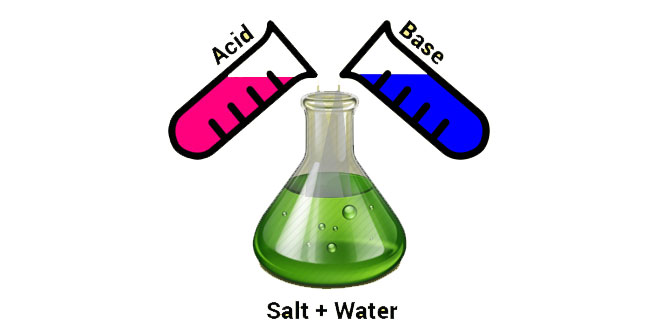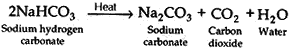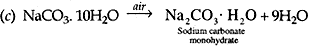Friday , September 25 2020# Acids, Bases and Salts: 10th Science

#### Question: Name the acid and based from which the following salts have been formed: (i) Sodium acetate. (ii) Ammonium chloride.

(i) Acid – CH3COOH (Acetic acid).
Base – NaOH (Sodium hydroxide).
(ii) Acid – HCl (Hydrochloric acid).
Base – NH4OH (Ammonium hydroxide).

#### Question: Write the chemical name of Na2CO3.10H2O and Na2CO3. Write the significance of 10H2O. Mention the term used for water molecules attached with a salt.

Answer: Na2CO3.10H2O – Hydrated salt / Sodium carbonate with water of crystallization (Sodium carbonate decahydrate).
Na2CO– Anhydrous salt / Sodium carbonate 10H2O means water of crystallization. It imparts colour and shape to the crystals.

#### Question: Crystals of a substance changed their colour on heating in a closed test tube but regained it after sometime when they were allowed to cool down. Name the substance and write its formula and explain the phenomenon involved.

Answer: Copper sulphate crystals (CuSO4. 5H2O). 5 molecules of water are present in one formula unit of copper sulphate. When heated it loses its water and salt turns white (becomes anhydrous).

#### Question: A compound ‘X’ is a constituent of baking powder. It is used as an antacid. When ‘X’ is heated it gives out a ‘Y’ which, when passed through lime water turns it milky and salt ‘Z’ is formed which is the main constituent of washing power. Identify X, Y and Z. Write balanced chemical equations for the reactions involved.

X – Sodium hydrogen carbonate (NaHCO3)
Y – Carbon dioxide (CO2)
Z – Sodium carbonate (Na2CO3).#### Question: The marble statues are often slowly corroded when kept in open for a long time. Give suitable reason.

Answer: Chemically, marble is calcium carbonate. All metal carbonates and hydrogen carbonates react with acids to give corresponding salt, carbon dioxide and water. Calcium carbonate reacts with acid to form calcium hydrogen carbonate, which is soluble in water and thus washed away. So the marble statues are slowly corroded.
CaCO3(s) + H2O(l) + CO2(g)  →  Ca(HCO3)2(aq)

#### Question: A solution of a substance ‘X’ is used for testing carbon dioxide. Identify ‘X’. What will be its reaction with carbon dioxide? Write a balanced equation for the reaction.

Answer: (i) X is lime water Ca(OH2).
(ii) Due to the formation of calcium carbonate, lime water turns milky when carbon dioxide gas is passed through it.
(iii) Ca(OH)+ CO→ CaCO+  H2O

#### Question: Write balanced chemical equations for the following: (1) Calcium carbonate reacts with hdrochloric acid. (2) Calcium oxychloride reacts with hydrochloric acid. (3) Sodium hydroxide reacts with zinc.

1. CaCO+ 2HCl  → CaCl+  H2O + CO2
2. 2CaOCl2+ 4HCl   →    2CaCl2  + 2H2O + 2Cl2
3. 2NaOH + Zn → Na2ZnO+ H2

#### Question: Identify A, B and C in the following reactions and write the chemical name of A, B, C. (1) HNO3  + NaOH →   A + H2O (2) B + Ca(OH)2  → CaSO4  + 2H2O (3) HCl + C → KCl + H2O

1. A: NaNO3 (Sodium nitrate)
2. B: H2SO4(Sulphuric acid)
3. C: KOH(Potassium hydroxide)

#### Question: Deepika prepares HCl gas in her school laboratory using certain chemicals. She puts both dry and wet blue litmus in contact with the gas. (1). Name the reagents used by Deepika to prepare HCl gas. (2). State the colour changes observed with the dry and wet blue litmus paper. (3). Show the formation of ions when HCl gas combines with water.

1. The reagents used by Deepika to prepare HCl gas are sodium chloride and conc. sulphuric acid.
2. There is no colour change observed with dry litmus paper. However, wet blue litmus paper turns red.
3. HCl + H2O → H3O+ +  Cl

#### Question: (1) State the purpose of developing pH scale. (2) Mention the pH range for acids. (3) What is the pH of acid rain and how it affects the aquatic?

1. The purpose of developing pH scale was to quantify the amount of Hor OH– ions in a solution.
2. The pH range for acids is 0 – 7.
3. When pH of rain water is less than 5.6, it is called acid rain. It is lowers the pH of river water. Thus, the survival of aquatic life becomes difficult.

#### Question: (1) If we take hydrochloric acid and acetic of same concentration, which one will produce less H+ ion concentration? Out of two which one is weak acid? (2) If someone is suffering from acidity, which of the following would you suggest in order to cure it: Vinegar, orange juice, baking soda solution? Give reason for your answer.

1. (i) Out of the hydrochloric acid and acetic acid of same concentration, acetic acid will produces less Hions.
(ii) Acetic acid.
2. If someone is suffering from acidity, I would suggest baking soda solution to cure it because it neutralises the excess acid produced in the stomach and provides relief.

#### Question: Give three practical applications of neutralisation reaction.

1. Antacids have been developed on the basis of neutralisation reaction.
2. Tooth pastes are basic in nature which neutralise the excess of acid produced in our mouth.
3. Milkman adds a very small amount of baking soda to milk to neutralise the lactic acid produced in the milk.

#### Question: Write chemical name and formula of the following: (1) Salt formed by the combination of weak acid like carbonic acid and strong base like sodium hydroxide. (2) Basic substance formed by chlor – alkali process. (3) Substances added in cakes to make them fluffy.

1. Sodium carbonate (Na2CO3).
2. Sodium hydroxide (NaOH).
3. Sodium hydrogen carbonate (NaHCO3).

#### Question: Give the chemical name formula of: (1) Soda ash (2) Blue vitriol (3) Baking soda

1. Anhydrous sodium carbonate; Na2CO3
2. Copper sulphate crystals; CuSO4.5 H2O
3. Sodium hydrogen carbonate; NaHCO3

#### Question: Write balanced chemical equations for the reaction of dil HCl with: (1) Zn metal (2) Na2CO3 (3) NaOH

1. Zn + 2HCl → ZnCl+H2
2. Na2CO3 + 2HCl → 2NaCl + CO2
3. NaOH + HCl →  NaCl + H2O

#### Question: (1) Name the products obtained in chlor-alkali process. (2) Name the gases liberated at anode and at cathode respectively. (3) Name the solution which is used as raw material in this process.

1. Chlorine gas, hydrogen gas and sodium hydroxide solution.
2. Chlorine gas is given off at the anode and hydrogen gas at the cathode.
3. Brine solution (common salt).

#### Question: Complete and balance the following equations: (a) Ca(OH)2 + Cl2 → (b) CaSO4. ½ H2O + H2O → (c) Na2CO3. 10H2O →

(i) Ca(OH)+ Cl→ CaOCl+ H2O
(ii) CaSO4. ½ H2O + ½ H2O → CaSO4.2H2O#### Question: (i) What do you understand by the term hydrated salt? (ii) Give two examples of hydrated salt which are white and state their chemical formula.

1. The salts having water of crystallisation with them are known as hydrated salts. Some salts unite with a definite quantity of water while crystallising out from their solutions, and this is known as the water of crystallisation.
2. (a)Sodium sulphate decahydrate (Na2CO3.10H2O). It is commonly known as washing soda.
(b) Hydrated calcium sulphate (CaSO4.2H2O). It is commonly known as gypsum.

#### Question: (1). Give the chemical names of acids present in: (i) ants   (ii) lemon    (iii) milk    (iv) tomato (2). Write the chemical names of two salts belonging so sodium family.

1. (i) formic acid/methanoic acid
(ii) citric acid
(iii) lactic acid
(iv) oxalic acid
2. (i) Sodium carbonate (Na2CO3)
(ii) Sodium sulphate (Na2SO4)

#### Question: Explain why: (1) Colour of copper sulphate crystals changes on heating? (2) baking soda acts as an antacid? (3) an acid should be added to water diluting?

1. It loses its 5 molecules of water of crystallisation hence colour changes.
2. It is alkaline and neutralises excess acid to relieve pain.
3. As dilution of acids is an exothermic reaction and adding water to acids will splash and cause burns.